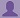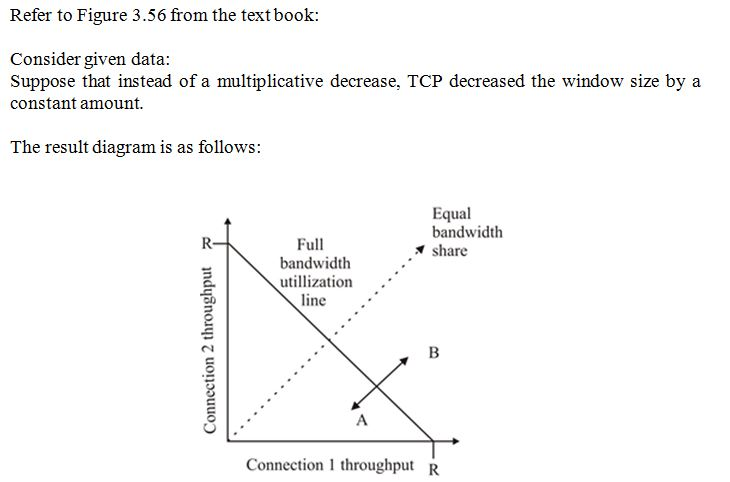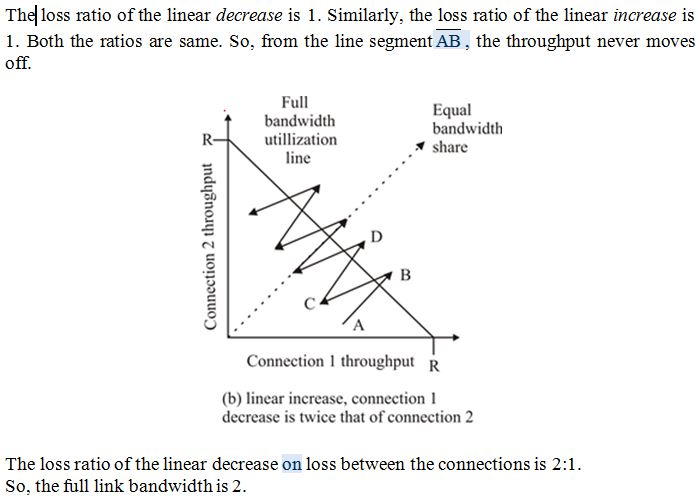To start with, Sr2Jr’s first step is to reduce the expenses related to education. To achieve this goal Sr2Jr organized the textbook’s question and answers. Sr2Jr is community based and need your support to fill the question and answers. The question and answers posted will be available free of cost to all.

# Computer Networking : A Top-down Approach

 Authors: James F. Kurose, Keith W. Ross ISBN: 9780132856201 Edition: 6
 Chapter: Transport Layer Exercise: Problems Question: 41

###Question

Refer to Figure 3.56, which illustrates the convergence of TCP’s AIMD algorithm. Suppose that instead of a multiplicative decrease, TCP decreased the window size by a constant amount. Would the resulting AIAD algorithm converge to an equal share algorithm? Justify your answer using a diagram similar to Figure 3.56.

###Answer### Discussions

Post the discussion improve the above solution.